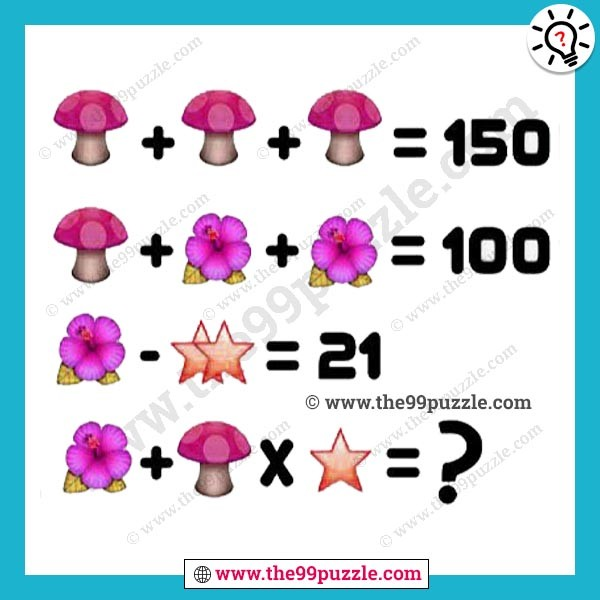# Mushroom Picture Puzzle with answer – Puzz158

Mushroom Picture Puzzle for the genius mind. These picture puzzles are challenging for people. Can you calculate the math equation picture puzzle? Math logical reasoning questions can help you to quick solving. Most people fail to answer this math logic puzzle.Mushroom = 50

Jaba Flower = 25

Star = 2

50+50+50=150

50+25+25=100

25-(2+2)=21

25+50×2=125